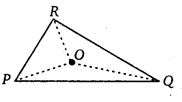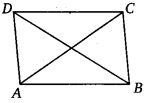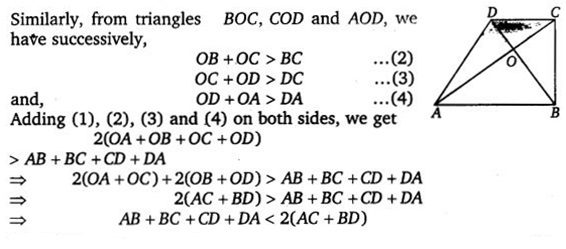# Class 7 Maths NCERT Solutions for Chapter – 6 The Triangle and its Properties Ex – 6.4

### The Triangle and its Properties

Question 1.
Is it possible to have a triangle with the following sides?
(i)
2 cm, 3 cm, 5 cm
(ii) 3 cm, 6 cm, 7 cm
(iii) 6 cm, 3 cm, 2 cm

Solution:
(i) Since, 2 + 3 > 5
So the given side lengths cannot form a triangle.
(ii) We have, 3 + 6 > 7, 3 + 7 > 6 and 6 + 7 > 3
i. e., the sum of any two sides is greater than the third side.
So, these side lengths form a triangle.
(iii) We have, 6 + 3 > 2, 3 + 2$\ngtr$ 6
So, the given side lengths cannot form a triangle.

Question 2.
Take any point O in the interior of a triangle PQR. Is(i) OP + OQ > PQ?
(ii) OQ + OR > QR ?
(iii) OR + OP > RP ?

Solution:
(i) Yes, OP + OQ > PQ because on joining OP and OQ, we get a ∆OPQ and in a triangle, sum of the lengths of any two sides is always greater than the third side.

(ii) Yes, OQ + OR > QR, because on joining OQ and
OR, we get a ∆OQR and in a triangle, sum of the length of any two sides is always greater than the third side.(iii) Yes, OR + OP > RP, because on joining OR and OP, we get a ∆OPR and in a triangle, sum of the lengths of any two sides is always greater than the third side.

Question 3.
AM is median of a triangle ABC. Is AB + BC + CA > 2AM?
(Consider the sides of triangles ∆ABM and ∆AMC.)Solution:
Using triangle inequality property in triangles ABM and AMC, we have
AB + BM > AM …(1) and, AC + MC > AM …(2)
Adding (1) and (2) on both sides, we get
AB + (BM + MC) + AC > AM + AM
⇒ AB + BC + AC > 2AM

Question 4.
ABCD is a quadrilateral. Is AB + BC + CD + DA > AC + BD?Solution:

Question 5.
ABCD is a quadrilateral. Is AB + BC + CD + DA < 2(AC + BD)?

Solution:
Let ABCD be a quadrilateral and its diagonals AC and BD intersect at O. Using triangle inequality property, we have
In ∆OAB
OA + OB > AB ……(1)Question 6.
The lengths of two sides of a triangle are 12 cm and 15 cm. Between what two measures should the length of the third side fall?

Solution:
Let x cm be the length of the third side.
Thus, 12 + 15 > x, x + 12 > 15 and x + 15 > 12
⇒ 27 > x, x > 3 and x > -3
The numbers between 3 and 27 satisfy these.
∴ The length of the third side could be any length between 3 cm and 27 cm

#### Class 7 Maths NCERT Solutions for Chapter – 6 The Triangle and its Properties Ex – 6.5

Lorem ipsum dolor sit amet, consectetur adipiscing elit. Phasellus cursus rutrum est nec suscipit. Ut et ultrices nisi. Vivamus id nisl ligula. Nulla sed iaculis ipsum.

Company Name